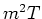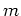Next: Steady, 1-D Body, Rectangular Up: Green's Function Solution Equation Previous: Heat Equation, General Case

# Laplace and Helmholtz Equation (Steady)

In this section the Green's Function Solution Equation is given for steady heat conduction. When the termis present (sometimes called the ``fin'' term) the steady heat conduction equation is a Helmholtz equation (with imaginary eigenvalues for real); otherwise it is a Laplace equation.

Subsections

2004-01-31